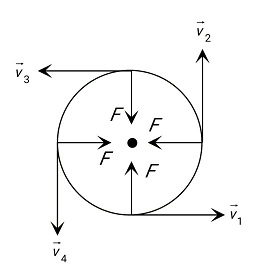Q

# I have a doubt, kindly clarify. A uniform rod of length l is being rotated in a horizontal plane with a constant angular speed about an axis passing through one of its ends. If the tension generated in the rod due to rotation is T(x) at a distance x f

A uniform rod of length l is being rotated in a horizontal plane with a constant angular speed about an axis passing through one of its ends. If the tension generated in the rod due to rotation is T(x) at a distance x from the axis, then which of the following graphs depicts it most closely ?

• Option 1)• Option 2)• Option 3)• Option 4)Views

Centripetal Force -F = Centripetal force

Angular velocity

n = frequency

- wherein

Force acts on the body along the radius and towards centre.This equation follows graph(1)

Option 1)Option 2)Option 3)Option 4)Exams
Articles
Questions